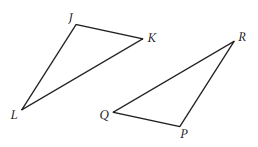Scan QR code or get instant email to install app

Question:

# Triangle JKL and triangle PQR are shown above. If ∠J is congruent to ∠P, which of the following must be true in order to prove that triangles JKL and PQR are congruent?A ∠L ≅ ∠R and JL = PR
explanation

If two angles and the included side of one triangle are congruent to corresponding parts of another triangle, the triangles are congruent. Since angles J and L are congruent to angles P and R, respectively, and the side lengths between each pair of angles, JL and PR, are also equal, then it can be proven that triangles JKL and PQR are congruent.